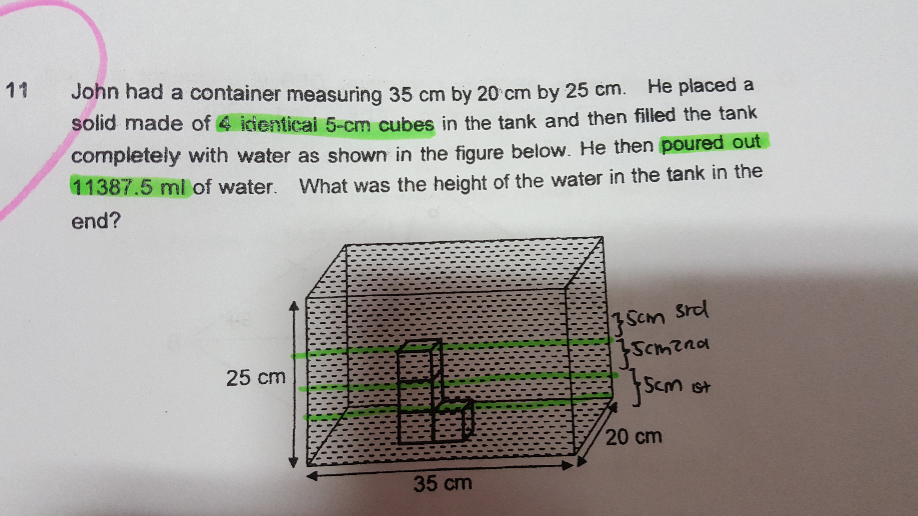Questionvolume of tank ——- 35 x 20 x 25 = 17500
volume of 4 identical 5-cm cubes ——- 4 x 5 x 5 x 5 = 500
17500 – 500 = 17000 (volume of water when tank is completely filled)
17000 – 11387.5 = 5612.5 (water in tank after 11387.5 ml is poured out)
35 x 20 x 5 – 2 x 5 x 5 x 5 = 3500 – 250 = 3250 (amount of water at height of 5 cm)
5612.5 – 3250 = 2362.5
35 x 20 x height – 5 x 5 x height ——- 2362.5
height ——- 2362.5/(35 x 20 – 5 x 5) = 3.5
3.5 + 5 = 8.5

Ans : 8.5 cm.

Hi tay,

May i asked, why only calculate with 1 cube height instead of 3 cubes ?

35 x 20 x 5 – 2 x 5 x 5 x 5 = 3500 – 250 = 3250 (amount of water at height of 5 cm)

Thank you.

Hi atm, note that the 4 cubes were stacked as follows :

top ——- 1 ( height : 10 to 15 cm)

middle ——- 1 cube (height : 5 to 10 cm)

bottom ——– 2 cubes (height : 1 to 5 cm)

Thus, when the height of the water level was at 5 cm, only the 2 cubes lined side by side at the bottom, with height of 5 cm,  should be considered in the calculations.